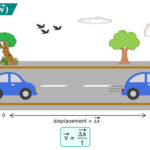Home / Physics / Principle of Superposition

# Principle of Superposition

The principle of superposition states that when two or more waves overlap in space, the resultant disturbance is the sum of their individual disturbances. A necessary condition for the superposition principle is that the amplitudes must be small such that the waves do not scatter or change form.

Superposition is an important concept as it can explain many phenomena in physics. The interference and diffraction of waves and standing waves are based on the superposition principle. This principle holds for all kinds of waves, like sound waves, light waves, electromagnetic waves, and surface waves.

## Superposition Principle and Interference of Waves

When we talk about disturbances in waves, we refer to the displacement in a vertical plane. Since displacement can be positive or negative, the net displacement can either be greater or less than the individual wave displacements. When both displacements are of the same sign, the resulting interference is called constructive interference. When both displacements have opposite signs, the resulting interference is called destructive interference. Constructive and destructive interferences are illustrated in the image below.

## Superposition Principle Equations

A function F(x) that satisfies the superposition principle is a linear function. In simple mathematical notation, it is written as

F (x1 + x2) = F (x1) + F (x2)

Consider two waves whose displacements are given by y1 and y2. Then, according to the principle of superposition, the net displacement y is given by

y = y1 + y2

The above equation can be generalized to n number of waves whose displacements are y1, y2, y3,…,yn. In such case, the net displacement is given by

y = y1 + y2 + y3 + … + yn

For the above equation to be valid, y1, y2, y3, …, yn must be linear.

Suppose the displacement is a function of the position x. Then, y is written as y ≡ y (x). The superposition of the two functions y1 (x) and y2 (x) is given by

y (x) = y1 (x) + y2 (x)

Consider a wave traveling along a stretched string with amplitude A, frequency ω, and wave number k. Its time-dependent displacement is given by

y1 (x, t) = A sin (kω – t)

Another wave traverses along the string but is shifted by a phase ø. Its displacement is given by

y2 (x, t) = A sin (kω – t + ø)

The two waves are coherent. Let us apply the superposition principle to combine these two waves and obtain the net displacement.

y (x, t) = y1 (x, t) + y2 (x, t)

=> y (x, t) = A [sin (kω – t) + sin (kω – t + ø)]

=> y (x, t) = 2A cos (ø/2) · sin (kω – t + ø/2)

Like its constituents, the resultant wave is sinusoidal, traveling in the positive x-direction with an amplitude 2A cos (ø/2). Its phase angle is half the phase difference of its constituent waves.

## Example Problems

Problem 1: Two light waves are represented by y1(t) = 5 sin(ωt) and y2(t) = 2 sin (ωt + π/2). What will be the resultant amplitude of superposition?

Solution

Given y1(t) = 5 sin(ωt) and y2(t) = 2 sin (ωt + π/2) = 2 cos (wt)

The resultant displacement is

y(t) = y1(t) + y2(t)

=> y(t) = 5 sin(ωt) + 2 cos (wt)

The resultant amplitude is

A = √(52 + 22) = 5.39

Article was last reviewed on Monday, January 2, 2023

### Related articlesLaminar FlowRadius of GyrationNewton’s Law of CoolingVelocity